### MEMO 2014 pojedinačno problem 3

Kvaliteta:
Avg: 2,5
Težina:
Avg: 4,7
Let$ABC$ be a triangle with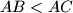$AB < AC$ and incentre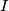$I$. Let$E$ be the point on the side$AC$ such that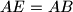$AE = AB$. Let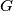$G$ be the point on the line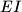$EI$ such that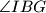$\angle IBG$ =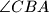$\angle CBA$ and such that$E$ and$G$ lie on opposite sides of$I$.
Prove that the line$AI$, the perpendicular to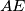$AE$ at$E$, and the bisector of the angle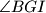$\angle BGI$ are concurrent.
Izvor: Srednjoeuropska matematička olimpijada 2014, pojedinačno natjecanje, problem 3